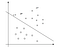# Support Vector Machine Math for people who don’t care about optimization

I long avoided the math behind Support Vector Machines because I know it's an optimization problem, and unless I am minimizing something with gradient descent, I don’t know much about optimization or linear programming or any of that.

However, I spent some time reading up on optimization finally and hope to make the concepts by SVM math more accessible for people with little optimization background.

First, let's discuss what it is we are trying to do. Consider the following plot:Image 1: Example Hyperplane Solved For Using an SVM. Figure Credit: Andrew Ng

The line separating the X’s from the O’s is called the separating hyperplane, where a hyperplane is just a plane…

# Intuition — Why L1 Regularization Pushes Coefficients to 0

I always hear a general hand-wavey statement that “Ridge Regression pushes coefficients towards zero, but Lasso Regression actually zeros out coefficients”… but why? Looking at the math didn’t make this statement immediately obvious for me so I wanted to write out an explicit example.

Suppose we have the following dataset

Where I will now refer to NQ as neighborhood quality, SQ as school quality, and HP as housing price.

We quickly notice that we can predict the price of a house perfectly with the function 2×HP=NQ. That is, we don’t even need to know anything about school quality.

When formulating…

# Nonlinear Regression Tutorial with Radial Basis Functions

Let's take a look at basis function regression which allows us to model non-linear relationships. If you are familiar with regular linear regression, then you know the goal is to find parameters (α,β) such that we can find the line of best fit y=αx+β.

When performing non-linear regression, we are no longer just solving for an equation of a line. Now, our high-level goal is to solve for the best linear combination of a set of basis functions that allows us to model something non-linear.

In other words, imagine we have some simple dataset

Now, suppose we have a set…

# SEMBLEU: A Robust Metric for AMR Parsing Evaluation

I have recently begun exploring Abstract Meaning Representations for Semantic Inference. I am familiar with the popular SMATCH metric but today will learn about SEMBLEU, which is a more robust evaluation for AMR parsers.

# AMR

The whole point of an AMR graph is to encode the semantics of a sentence into a directed graph. Nodes in the graph represent semantic terms in the sentence and the edges identify the semantic relations between nodes. A major goal in semantic inference tasks is to be able to extract these graphs from regular sentences. …## Joseph Gatto

Machine Learning Ph.D. Student